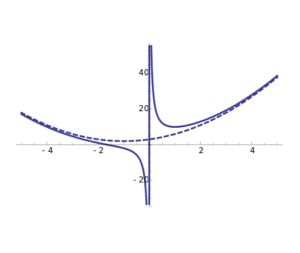Home -> Solved problems -> Curvilinear asymptote

## Find out how to find the equation of a rational function curvilinear asymptote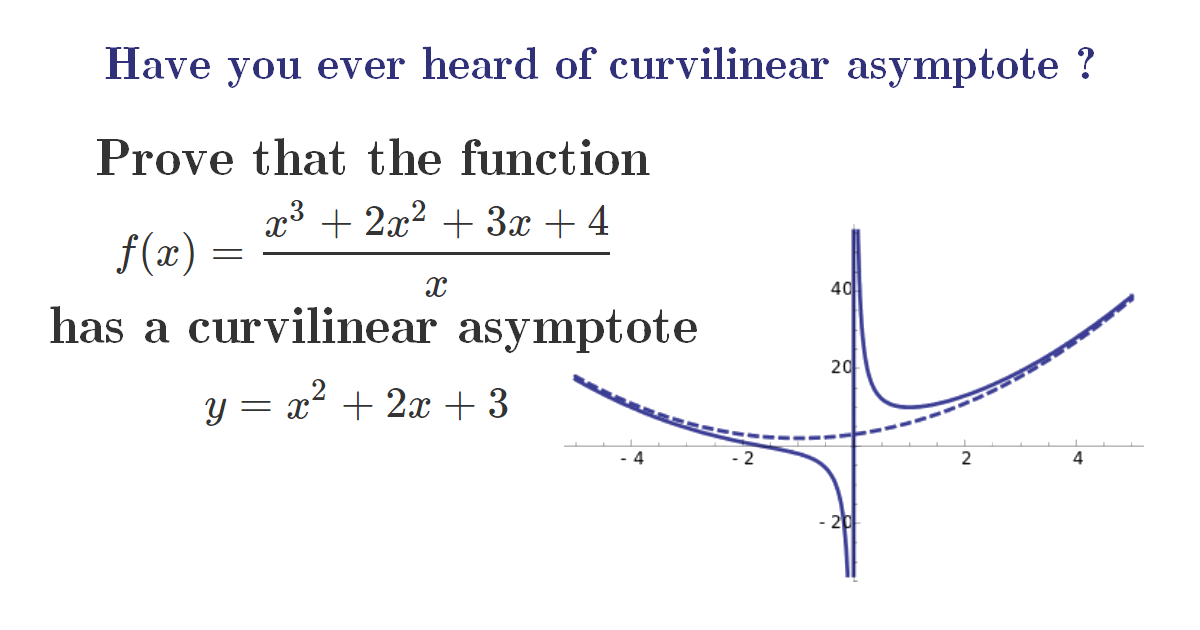### Solution

$$f$$ is a function defined on $$]-\infty, 0[\cup] 0, \infty[$$ by: $f(x)=\frac{x^{3}+2 x^{2}+3 x+4}{x}$ We are interested in studying $$f$$ as $$x$$ approaches infinity ($$-\infty$$ and $$\infty$$)
\begin{aligned} \lim _{x \rightarrow\infty} f(x) &=\lim _{x \rightarrow\infty} \frac{x^{3}+2 x^{2}+3 x+4}{x} \\\\ &=\lim _{x \rightarrow\infty} \frac{x^{3}\left(1+\frac{2}{x}+\frac{3}{x^{2}}+\frac{4}{x^{3}}\right)}{x} \\\\ &=\lim _{x \rightarrow\infty} x^{2}\left(1+\frac{2}{x}+\frac{3}{x^{2}}+\frac{4}{x^{3}}\right) \\\\ &=\lim _{x \rightarrow\infty} x^{2}=\infty \end{aligned} Now, let’s study the behavior of $$f$$ relative to $$x$$ as $$x$$ approaches $$\infty$$ \begin{aligned} \lim _{x \rightarrow\infty} \frac{f(x)}{x} &=\lim _{x \rightarrow\infty} \frac{x^{3}+2 x^{2}+3 x+4}{x^{2}} \\\\ &=\lim _{x \rightarrow\infty} x\left(1+\frac{2}{x}+\frac{3}{x^{2}}+\frac{4}{x^{3}}\right) \\\\ &=\lim _{x \rightarrow\infty} x=\infty \end{aligned} Therefore, $$f$$ has no slant asymptote when $$x$$ approaches $$\infty$$. Thus, we increase the degree of power of $$x$$ and we study the behavior of the function $$f$$ relative to $$x^{2}$$ as $$x$$ approaches $$\infty$$ \begin{aligned} \lim _{x \rightarrow \infty} \frac{f(x)}{x^{2}} &=\lim _{x \rightarrow \infty} \frac{x^{3}+2 x^{2}+3 x+4}{x^{3}} \\\\ &=\lim _{x \rightarrow\infty} 1+\frac{2}{x}+\frac{3}{x^{2}}+\frac{4}{x^{3}} \\\\ &=1 \end{aligned} At this step, we know that we are dealing with a parabola with equation of general form: $$y=ax^{2}+bx+c$$, knowing that we have already find $$a=1$$ the equation becomes: $$y=x^{2}+bx+c$$. Now, let’s try to find $$b$$ and $$c$$ \begin{aligned} \lim _{x \rightarrow+\infty} \frac{f(x)-x^{2}}{x} &=\lim _{x \rightarrow \infty} \frac{\frac{x^{3}+2 x^{2}+3 x+4}{x}-x^{2}}{x} \\\\ &=\lim _{x \rightarrow \infty} \frac{x^{3}+2 x^{2}+3 x+4-x^{3}}{x^{2}} \\\\ &=\lim _{x \rightarrow \infty} \frac{2 x^{2}+3 x+4}{x^{2}} \\\\ &=\lim _{x \rightarrow \infty} \frac{x^{2}\left(2+\frac{3}{x}+\frac{4}{x^{2}}\right)}{x^{2}} \\\\ &=\lim _{x \rightarrow \infty} 2+\frac{3}{x}+\frac{4}{x^{2}} \\\\ &=2 \end{aligned} $\Rightarrow b=2$ Therefore, $y=x^{2}+2x+c$ Now, let’s try to find $$c$$ \begin{aligned} \lim _{x \rightarrow \infty} f(x)-x^{2}-2 x &=\lim _{x \rightarrow \infty} \frac{x^{3}+2 x^{2}+3 x+4}{x}-x^{2}-2 x \\\\ &=\lim _{x \rightarrow \infty} \frac{x^{3}+2 x^{2}+3 x+4-x\left(x^{2}+2 x\right)}{x} \\\\ &=\lim _{x \rightarrow \infty} \frac{x^{3}+2 x^{2}+3 x+4-x^{3}-2 x^{2}}{x} \\\\ &=\lim _{x \rightarrow \infty} \frac{3 x+4}{x} \\\\ &=\lim _{x \rightarrow \infty} \frac{x\left(3+\frac{4}{x}\right)}{x} \\\\ &=\lim _{x \rightarrow \infty} 3+\frac{4}{x}=3=c \end{aligned} For $$-\infty$$ we use the same steps as $$\infty$$ and we will find out that the parabola: $$y=x^{2}+2 x+3$$ is also a parabolic asymptote for the function $$f$$ but as $$x$$ approaches $$-\infty$$.  Let’s recap, $$f$$ has a curvilinear asymptote as $$x$$ approaches $$-\infty$$ and $$\infty$$ which is a parabolic asymptote $\huge y=x^{2}+2 x+3$
Home -> Solved problems -> Curvilinear asymptote

### Related Topics

Find the volume of the square pyramid as a function of $$a$$ and $$H$$ by slicing method.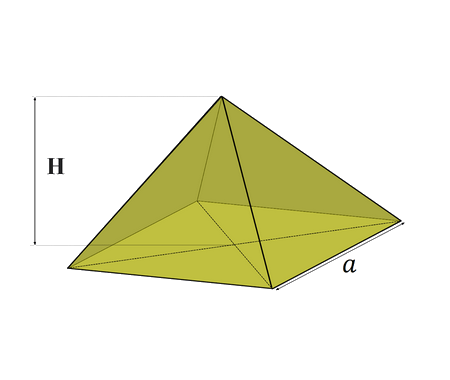Prove that $\lim_{x \rightarrow 0}\frac{\sin x}{x}=1$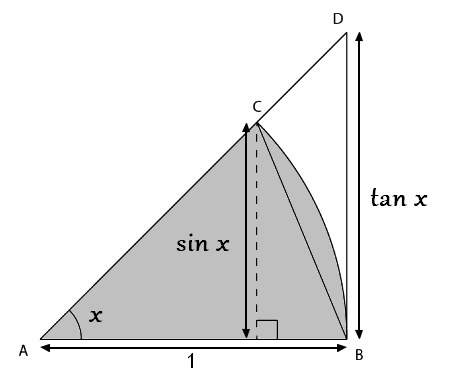Prove that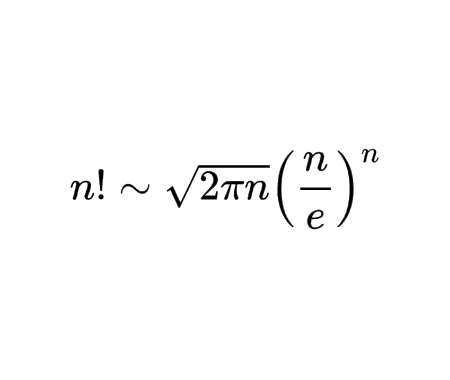Calculate the half derivative of $$x$$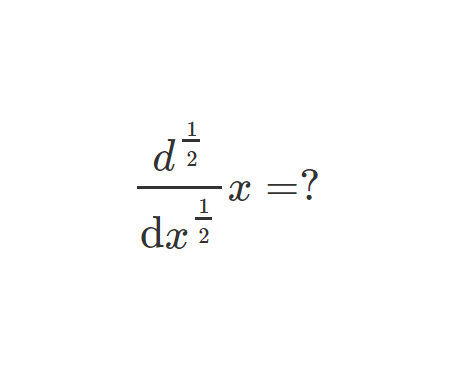Prove Wallis Product Using Integration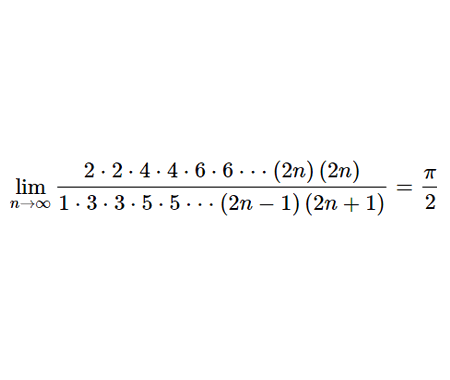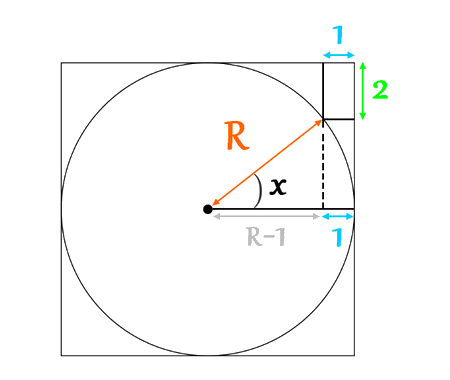Calculate the volume of Torus using cylindrical shells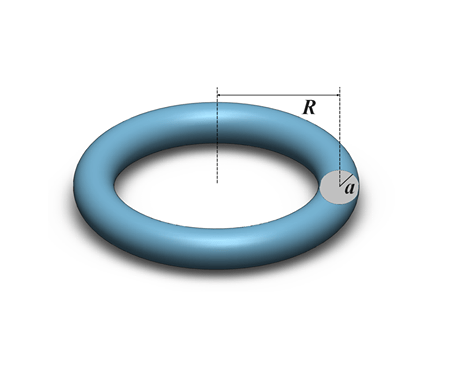Find the derivative of exponential $$x$$ from first principles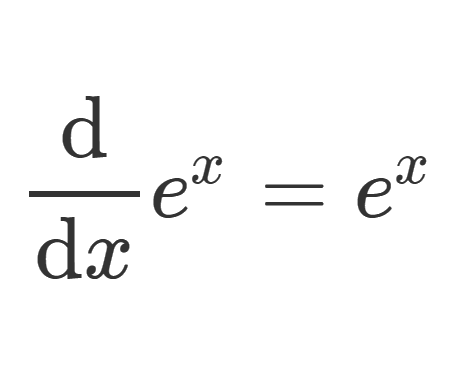Calculate the sum of areas of the three squares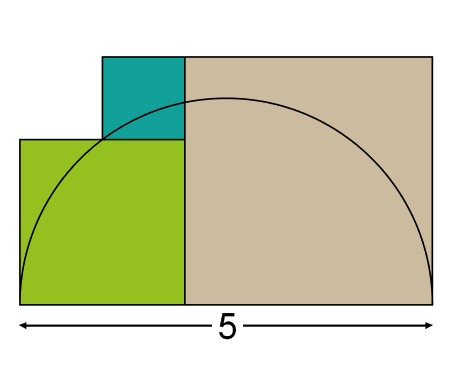Find the equation of the curve formed by a cable suspended between two points at the same height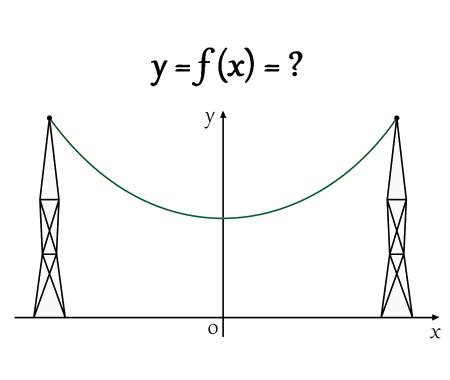Solve the equation for real values of $$x$$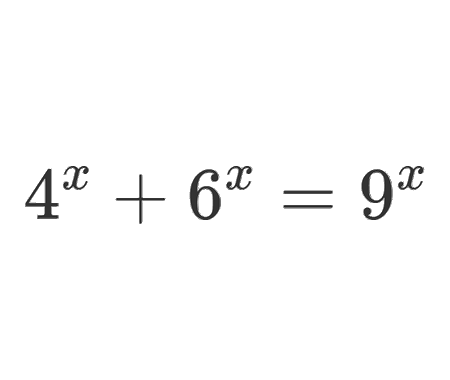Solve the equation for $$x\epsilon\mathbb{R}$$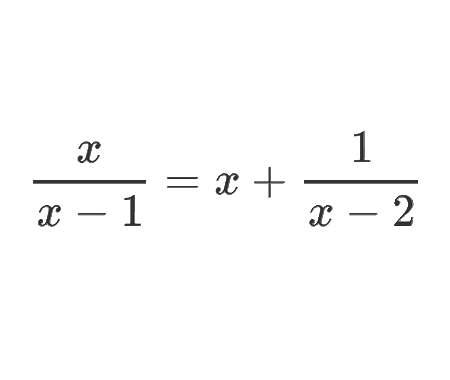Determine the angle $$x$$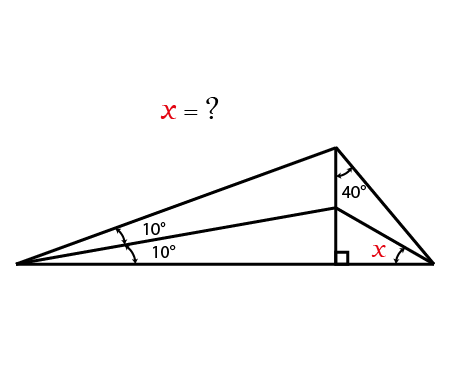Calculate the following limit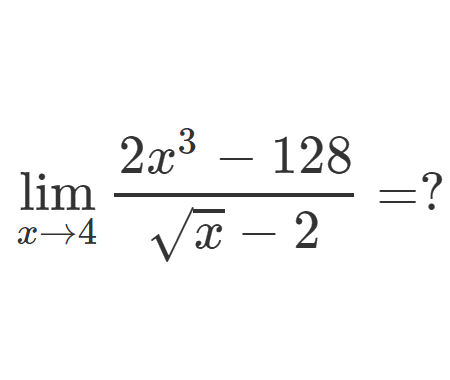Calculate the following limit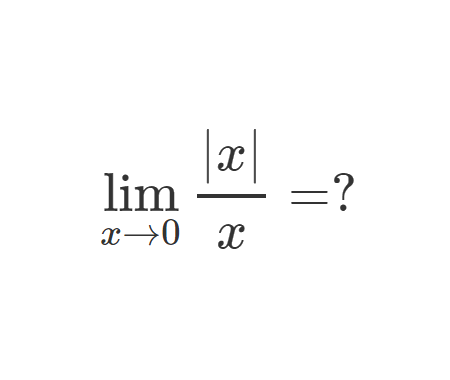Calculate the integral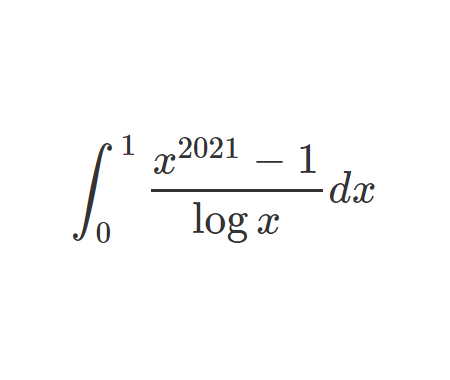Challenging problem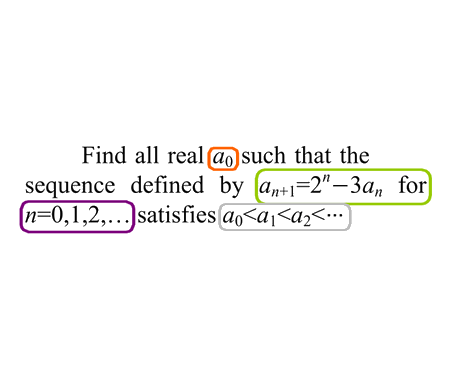Prove that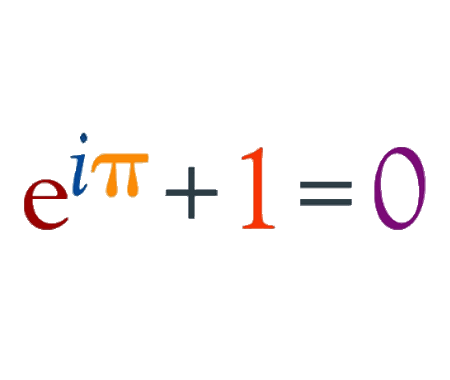Prove that $$e$$ is an irrational numberFind the derivative of $$y$$ with respect to $$x$$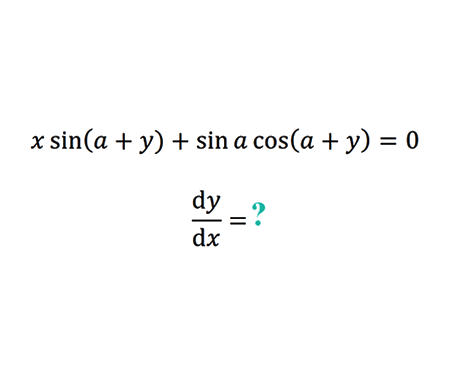Find the limit of width and height ratio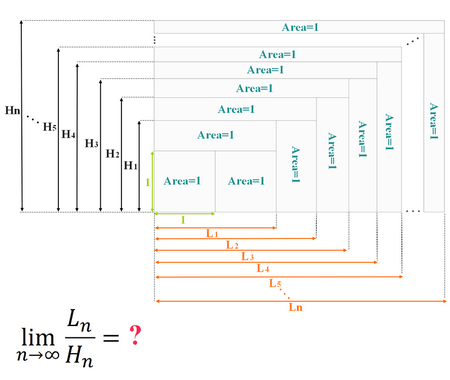How Tall Is The Table ?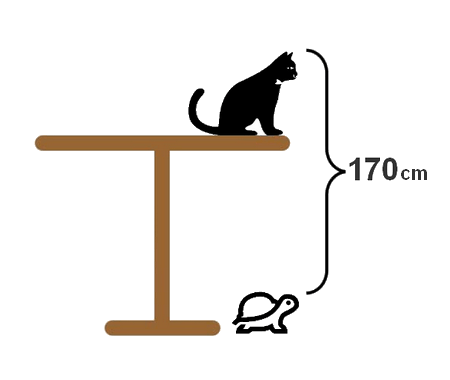Why 0.9999999...=1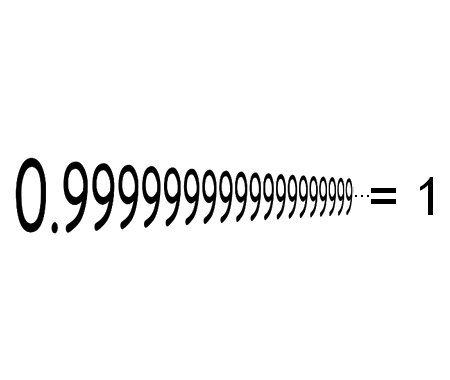Solve the equation for $$x \in \mathbb{R}$$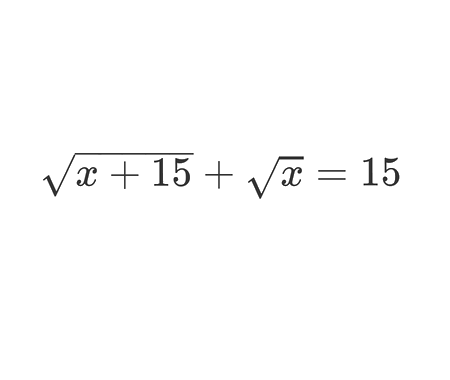Calculate the following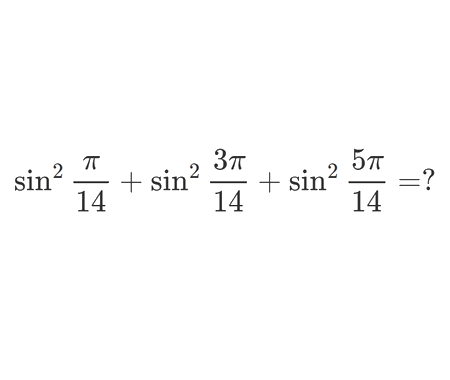Is $$\pi$$ an irrational number ?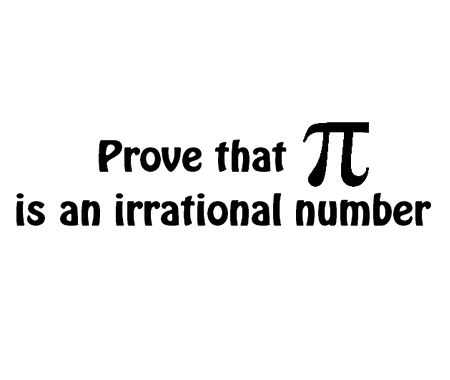How far apart are the poles ?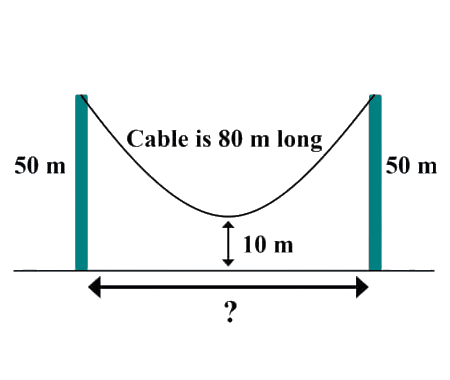Solve for $$x \in \mathbb{R}$$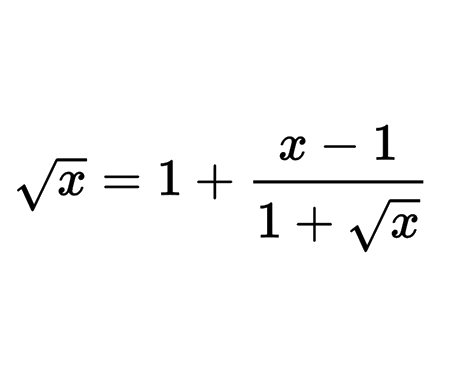What values of $$x$$ satisfy this inequality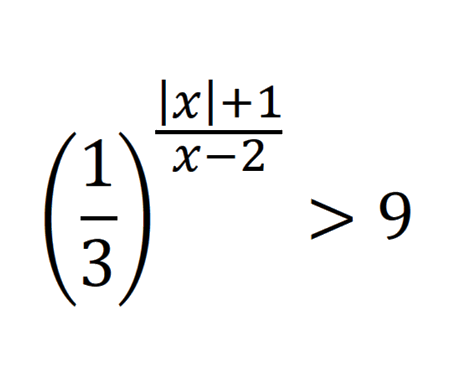Prove that the function $$f(x)=\frac{x^{3}+2 x^{2}+3 x+4}{x}$$ has a curvilinear asymptote $$y=x^{2}+2 x+3$$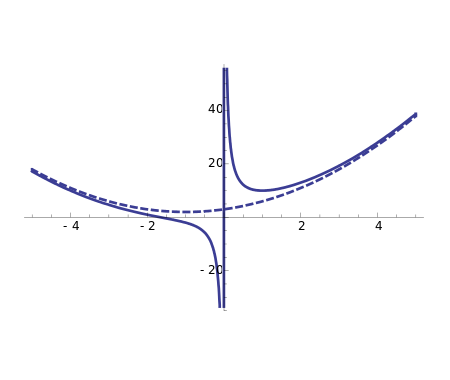Home -> Solved problems -> Curvilinear asymptote

#### Share the solution: Curvilinear asymptote